The script language OptiGrating 4.2 uses is a programming language based on the
BASIC language syntax. You use the script language to define the shape, chirp, and
apodization of a grating. To enter this definition, you have to choose the User Defined
option.

## Variables, Arrays, and Operators

Variables

A variable name can be any string beginning with a character. You cannot use special
characters and operators in the name. Some names are reserved for commands,
predefined constants, and functions. Variable names are case sensitive. For
example, ‘a’ is not the same as ‘A’; ‘Pos’ is not the same as ‘pos’ or ‘POS’.

Examples:

Valid variable names: a, pos, lambda, width1
Invalid variable names: *a, _pi, IF, RETURN

Note: Comment lines in the editing area are preceded by the double slash symbol //.

Arrays

You can use only one-dimensional arrays. You do not have to declare an array before
using it. For the array index, you can use a positive or a negative integer. The usage
format is array_name[index].

Examples:
A
B[-3]
a[x]=x*2

Mathematical Operators

OptiGrating supports the following standard mathematical operators. (listed in
decreasing priority):

^ power
* multiplication
/ division
– subtraction

Boolean Operators

OptiGrating supports the following standard Boolean operators.

= equal to
< less than
> more than
& and
| or
Examples:
A=b
A<b
B&c
C|d

Comparison Operators

The Comparison operators can be combined:

a<=b; a<>b; a=>b

## Commands and Statements: RETURN, IF, ERROR

All command and statement names must be in upper case letters.

Examples:

IF is a command
If or if can be a variable

The script language has a set of commands and control statements. However, not all
of them are needed to define user shape, chirp, and apodization. Therefore, here is a
list of the commands useful in OptiGrating.

The RETURN Command

The RETURN keyword terminates execution of the program in which it appears and
returns the control (and the value of expression, if given). Notice that if you don’t
specify RETURN in your program, the interpreter will return the value of expression
in the last logical order. You usually use the RETURN command if your program uses
IF – THEN commands.

Syntax: RETURN[expression]

Example 1:

A program with only one line (you don’t have to specify RETURN)

sin(3)*24+13*(2+6)

returns 107.38688. The same result is obtained from the program:

RETURN sin(3)*24+13*(2+6)

Example 2:

Consider the program:

cp = 100
a = Length-x
IF x<cp THEN
RETURN x
ELSE
RETURN a

The above program returns x if x is less than 100. Otherwise, it returns Length-x if x
is larger or equal than 100.

Example 3:

Let’s modify the previous example, Example 2, as follows:

cp = 100
a = Length-x
IF x<cp THEN
b = x
ELSE
b = a
RETURN b

This example gives the same result as Example 2. The difference is that another
variable b is used instead of returning the value of a directly.

The IF Statement

The IF statement controls conditional branching. The body of an IF statement is
executed if the value of the expression is nonzero. The syntax for the IF statement
has two forms.

Syntax1: IF expression THEN statement
Syntax2: IF expression THEN statement ELSE statement

In both forms of the IF statement, the expressions, which can have any value, are
evaluated, including all side effects.

In the first form of the syntax, if expression is nonzero (true), the statement is
executed. If expression is false, statement is ignored. In the second form of syntax,
which uses ELSE, the second statement is executed if expression is false. With both
forms, control then passes from the IF statement to the next statement in the program.

Examples:

The following are examples of the IF statement:

IF i > 0 THEN y = x / i ELSE x = i

In this example, the statement y = x/i is executed if i is greater than 0. If i is less than
or equal to 0, i is assigned to x.

For nesting IF statements and ELSE clauses, use BEGIN – END to group the
statements and clauses into compound statements that clarify your intent. If no
BEGIN – END are present, the interpreter resolves ambiguities by associating each
ELSE with the closest IF that lacks an ELSE.

IF i > 0 THEN         // Without BEGIN – END
IF j > i THEN
x = j
ELSE
x = i

The ELSE clause is associated with the inner IF statement in this example. If i is less
than or equal to 0, no value is assigned to x.

IF i > 0 THEN
BEGIN                   /* With BEGIN – END */
IF j > i THEN
x = j
END
ELSE
x = i

The BEGIN – END enclosing the inner IF statement in this example makes the ELSE
clause part of the outer IF statement. If i is less than or equal to 0, i is assigned to x.

The ERROR Keyword

The ERROR keyword stands for a constant and returns the error code (if any). In case
of a mathematical error, like division by zero or overflow, it will reset the error flag.

The constant returns the error code (if any) and in cases of mathematical errors
(division by zero, overflow, etc.) resets the error flag. This allows you to detect any
unexpected errors during calculation. The best example is to use the ERROR
command in the IF-THEN-ELSE statement.

Example 1:
The following one-line program

Length /x

results in the Error message: Division by zero, b=Length/x?????

This occurs because initially x has been set to 0.

Example 2:
Consider the program:

b=Length/x
IF ERROR THEN
b=100;
RETURN b

Now, whenever we have a mathematical error, b is set to 100 and the program will
continue without the error message.

ERROR is a constant, and you cannot assign any other value to it.

The FIRSTTIME Keyword

Flag – indicates FIRST time internal run.

Example:

S =7
IF FIRSTTIME THEN
RETURN 100
RETURN x*s

## Input dialog box

This dialog will appear if you use INPUT or GINPUT in User Defined Functions.

Syntax:

INPUT variable
INPUT “string” variable
INPUT “string” variable = init value
GINPUT variable=init value
GINPUT “string” variable = init value

Example INPUT

Usage of INPUT is limited. Because in OptiGrating you use user defined functions
usually in a loop, INPUT will ask you to input data every time you repeat the loop
(usually more than 100 times). To avoid this and to avoid redundant programming (IF
FIRSTTIME THEN …), use the function GINPUT (general input), which will ask you
for input data only the first time you enter the loop.

INPUT variable
INPUT “string” variable
INPUT “string” variable = init value

Example:

b=1
MAX = 8
INPUT “Factorial of:” MAX

……

You will get the same effect if you use:

b=1
INPUT “Factorial of:” MAX = 8

You will be asked to type a value in the Factorial Of box in the Input window.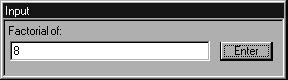Note: The initialization of MAX before INPUT is not necessary. If you delete the
line MAX = 8, the default value in the Input box will be 0.

Example GINPUT

GINPUT variable=init value
GINPUT “string” variable = init value

(general input)

Displays an Input window on the screen. (The text is optional.)
If the whole program (User Defined Function) is running more than once in the logical
(internal) block, GINPUT will ask you to enter data only the first time the program runs.

Example:

In OptiGrating, you will use the Apodization function :

s=4
tanh(s*(x/Length))*(tanh (s*(1-x/Length)))

Because each grating length is divided into a number of steps (let’s say 25), this
function will be called more than once per grating (25 times).

Note: Variable x will vary in each step: x(actual) = x(previous) + Length/25

In the Apodization function, you have one parameter “s” for changing the shape of the
apodization function. If you want to test different shapes, you will need to change the
value for each calculation. Instead of going through all dialog boxes and buttons to
reach the User Function dialog box, whenever you want to change “s”, you can use
the GINPUT function:

GINPUT “Input Shape parameter” s=4
tanh(s*(x/Length))*(tanh (s*(1-x/Length)))

You will be asked to input different “s” values each time you press the Calculation
button. Thus, you will be able to try different apodization shapes very quickly and
easily.

## Constants

Mathematical Constants

pi 3.14159265358979323846
e 2.71828182845904523536

Physical Constants

 _c 9979e8 m/s Speedoflightinfreespace _e 8542e-12 F/m Permittivityinfreespace _mi 4*pi*10e-7 H/m Permeabilityinfreespace _q 60219e-19 C Elementarycharge _me 1095e-31 kg Freeelectronmass _u 660531e-27 kg Atomicmassunit _mp 672614e-27 kg Protonrestmass _mn 674920e-27 kg Neutronrestmass _eV 60219e-19 J Energyunit(electron-volt) _h 626e-34 Js Planckconstant _hr 05459e-34 Js ReducedPlanckConstant _lc 4263096e-12 m Comptonwavelengthofelectron _Ry 13.6058 eV Rybergenergy _ri 09737312e7 1/m Rydbergconstant _kT 25.853 meV Thermalenergy _NA 022045e23 Avogadronumber _f 6486e4 C/mol Faradayconstant _a 297351e-3 Fine structureconstant _a0 2917715e-11 m Bohrradius _re 817939e-15 m Electronradius _mb 274096e-24 J/T Bohrmagnetron _kB 3807e-23 J/K Boltzmannconstant _sb 66961e-8 Stefan-Boltzmannconstant

## Functions

Commonly Used Functions

Other Functions

min(a,b) =a if a<b else b
max(a,b) =a if a>b else b
comb(n, k)= number of combinations for k object , n – return 0 total number of objects (n>=m),
if error
perm(m, n) =permutation mPn (0 if error)
gcd(a, b) = Greatest Common Divisor between a & b
lcm(a, b) = Largest Common Multiple between a & b
frc(x) = fractional part of ‘x’
int(x) = integer part of ‘x’
a>n0g alen(dx,y) Computes angle from Cartesian position (x,y), angle defined positive if y
negative if y < 0
randrand(MaxNum) => Randomize {0..MaxNum}

Fresnel Integral

fresnel_s(x) = Fresnel Integral SIN
fresnel_c(x) = Fresnel Integral COS
Evaluates the Fresnel integrals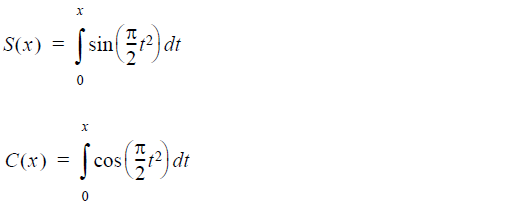fresnel_ffresnel_f(x) = function f(x) related to the Fresnel Integral
fresnel_g(x) = function g(x) related to the Fresnel Integral

Evaluates the functions f(x) and g(x) related to the Fresnel integrals by means of the
formulae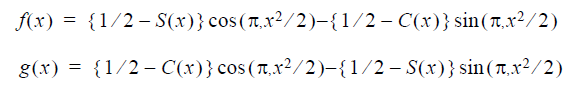Gamma Functions

Computes the value of the gamma function at xgamma(x)Gamma Function
lgamma(x)Natural logarithm of Gamma Function, x – must be positive

Error Function

erf(x)Error Function
erfc(x) Complementary Error Function”},

Computes the error function erf(x) and Complementary error function erfc(x)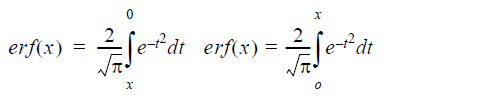When x>26 then erf(x)=1 and erfc(x)=0
When x< -5.5 then erf(x)=-1 and erfc(x)=2;

nexperfcNon exp erfc computes exp(x2)erfc(x)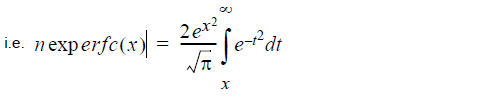Inverse error function y(x)

inverf(x,minx)

Evaluates the inverse error function y(x) where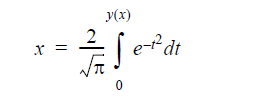x – it is necessary that -1 <x < 1
if |x| > 0.8 then value of x is not used in the procedure

minx    if |x| <= 0.8 then value minx is not used in the procedure
if |x|> 0.8 then minx has to contain the value of 1-|x|.

In the case that |x| is in the neighborhood of 1, cancellation of digits take place in the
calculation of 1-|x|

If the value 1-|x| is known exactly from another source, then minx has to contain this
value, which will give better results.

## Bessel Functions

Bessel function J

bessj0(x) – Bessel function J0(x) of the 1st kind of order 0
bessj1(x) – Bessel function J1(x) of the 1st kind of order 1
bessj [k] (x) – Bessel function Jk(x) of the 1st kind in order k

Bessel function Y (Weber’s function )

bessy0(x) – Bessel function Y0(x) of the 2nd kind of order 0
bessy0(x) – Bessel function Y1(x) of the 2nd kind of order 1
bessy [k] (x) – Bessel function Yk(x) of the 2nd kind of order k

Modified Bessel function I

bessi0(x) – Modified Bessel function I0(x) of the 1st kind of order 0
bessi1(x) – Modified Bessel function I1(x) of the 1st kind of order 1
bessi [k] (x) – Modified Bessel function Ik(x) of the 1st kind of order k

Modified Bessel function K

bessk1(x) – Mod. Bessel function K0(x) of the third kind of order 0
bessk1(x) – Mod. Bessel function K1(x) of the third kind of order 1
bessk [k] (x) – Mod. Bessel function Kk(x) of the third kind of order k

Spherical Bessel functions

spbessj[k](x) => Spherical Bessel funct. Jk+0.5(x)
Bessel function of the second kind (Neumann’s functions)
neumann(a,x) – Neumann function Ya(x) of the 2nd kind of order a
neumann1(a,x) – Neumann function Ya+1(x) of the 2nd kind of order a

Chebyshev Polynomials

chepol(n,x) – Computes the value of the Chebyshev polynomial Tn(x)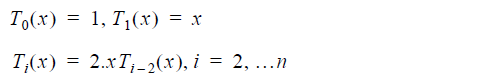## Conversions

Length

 ft_m ft_m(foot) Foottometer yd_m yd_m(yard) Yardtometer in_m in_m(inch) Inchestometer

Temperature

 c_k c_k(celsius) Celsius to Kelvin fh_k fh_k(farenheit) Farenheit to Kelvin fh_c fh_c(farenheit) Farenheit to Celsius k_c k_c(kelvin) Kelvin to Celsius c_fh c_fh(celsius) Celsius to Farenheit k_fh k_fh(kelvin) Kelvin to Farenheit

Other Conversions

 gal_l gal_l(gallon) Gallontoliter pt_l pt_l(pint) Pinttoliter oz_l oz_l(oz) Oztoliter in3_l in3_l(cubInc) CubicInchtoliter l_m3 l_m3(liter) Literto cubicmeter pd_kg pd_kg(pound) Poundtokilogram phe phe(wavelength[µm]) CalculatePhotonenergy[eV]